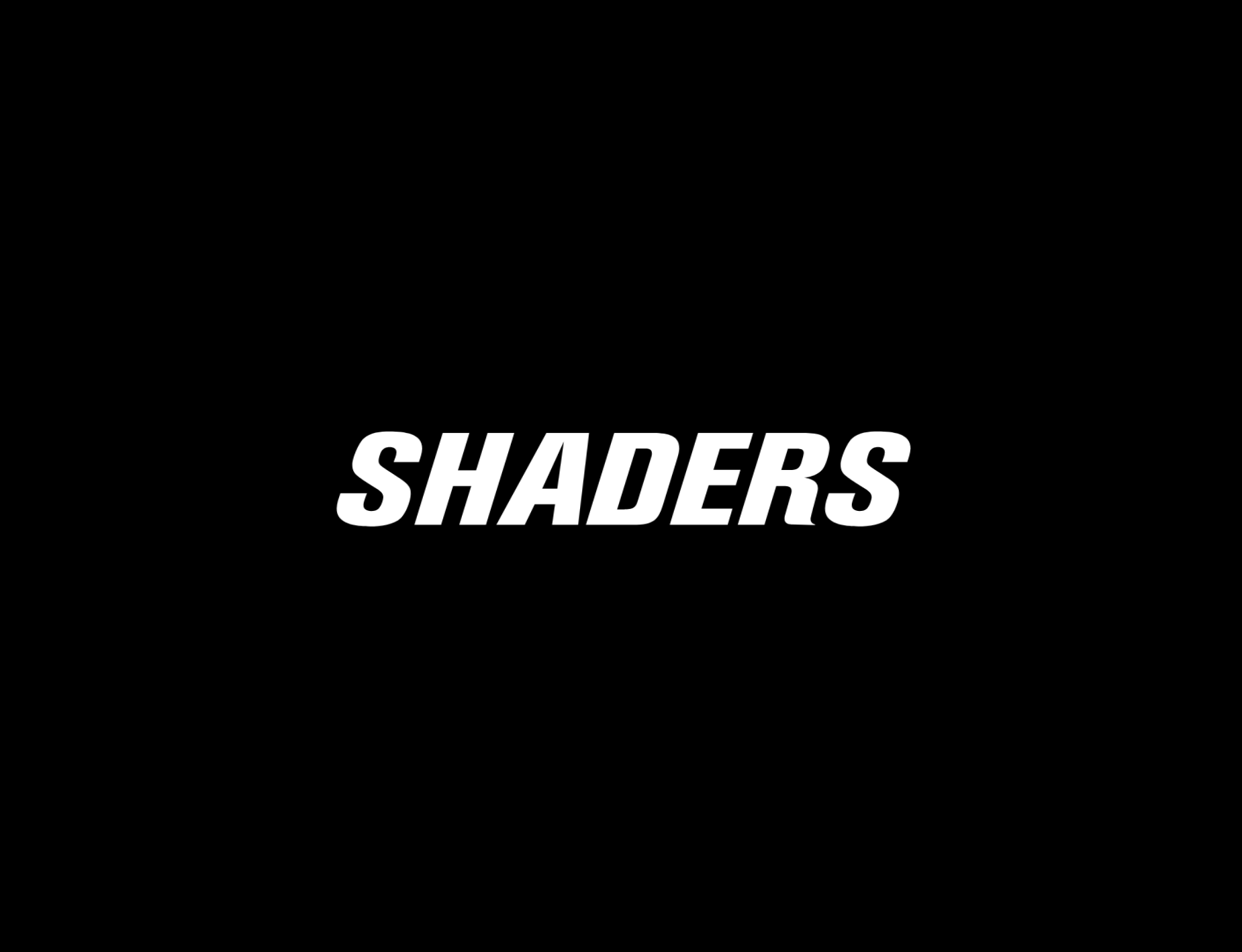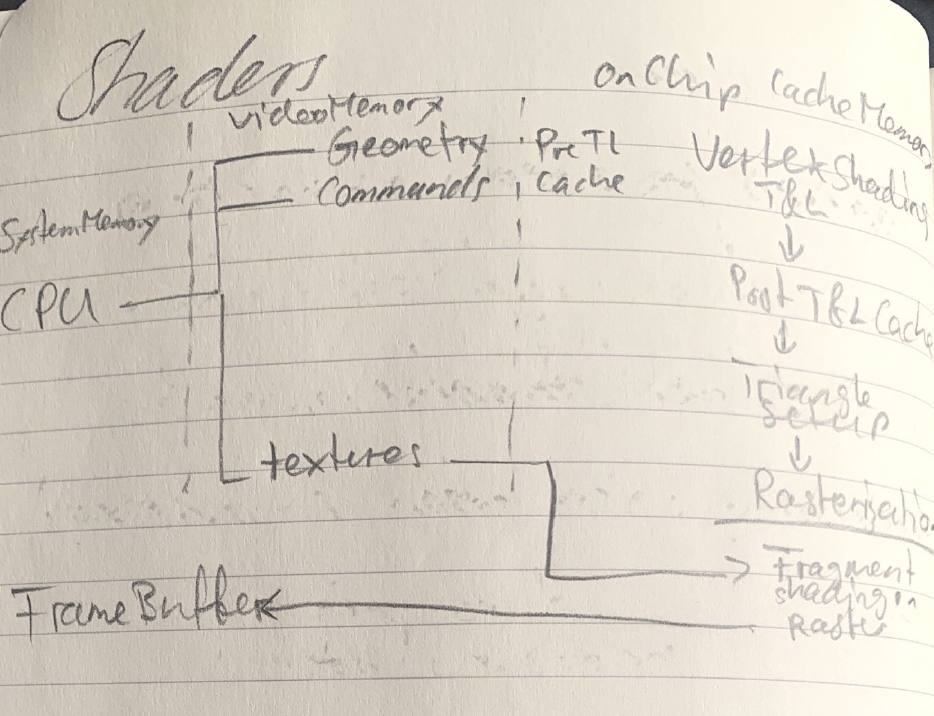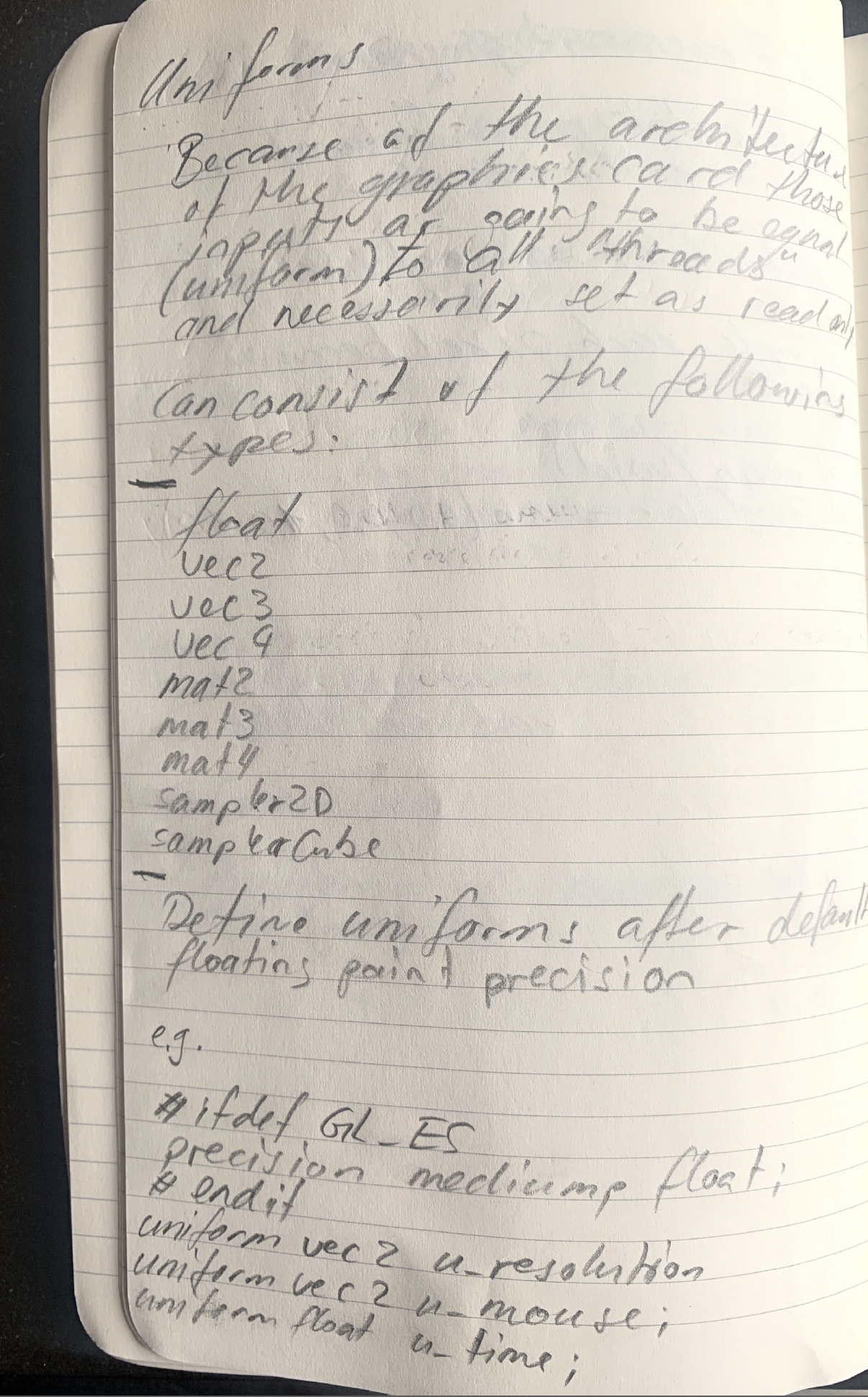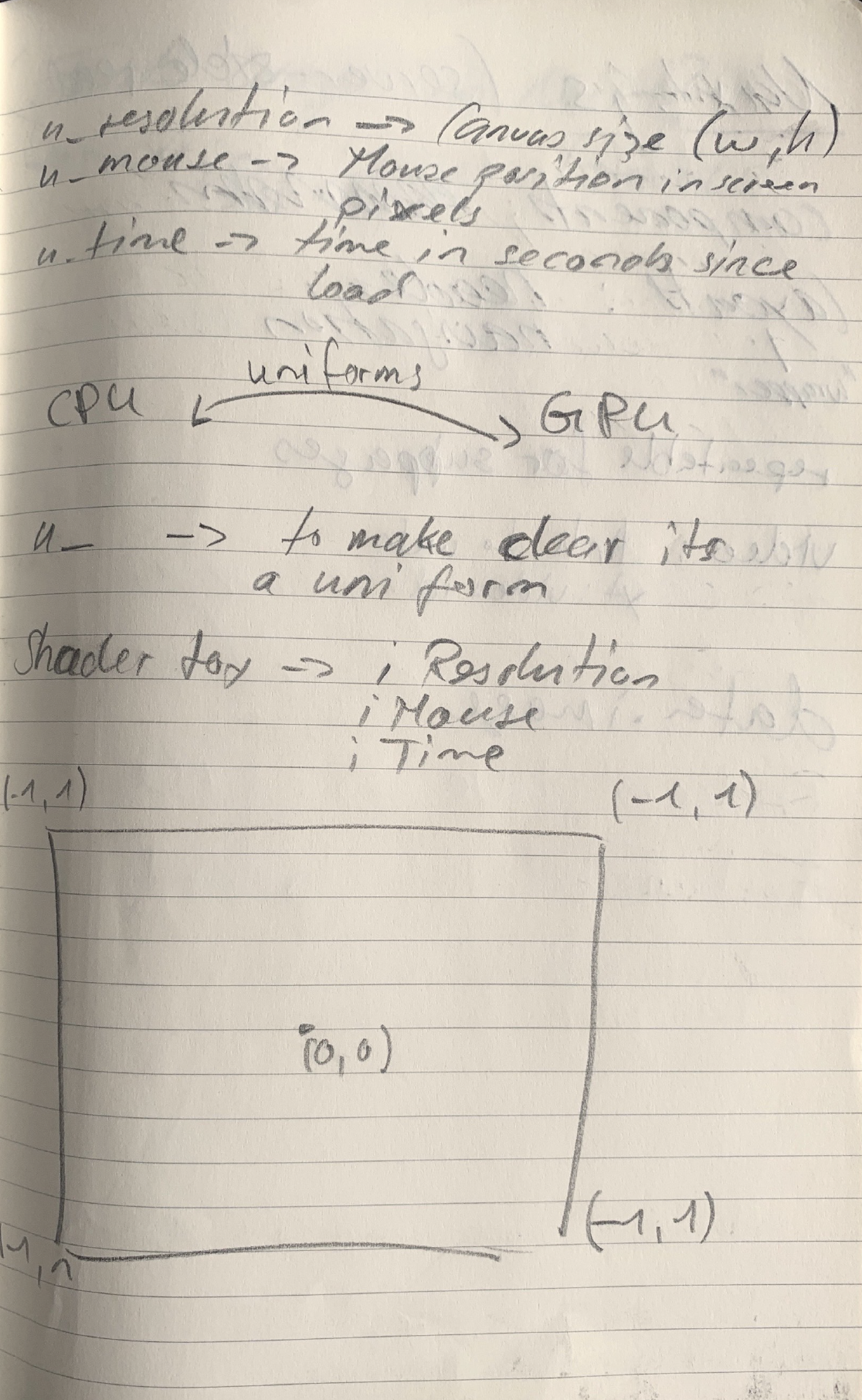webdevelopment: Alain Brusch
BackA shader normally involves the following structure:

```#version version_number
in type in_variable_name;
in type in_variable_name;

out type out_variable_name;

uniform type uniform_name;

void main()
{
// process input(s) and do some weird graphics stuff
...
// output processed stuff to output variable
out_variable_name = weird_stuff_we_processed;
}```The process we actually won't see:

1. Textures will be sent to GPU

2. Vertex Data (Position, Normals, Colors) transmitted

4. Fragment Shader calculates color values of each pixel of the triangles

- Controls position of vertices

- User defined lightning (per vertex)

- User defined Blending / shining

- Arbitrary Calculation (fish-eye)

- Acceleration of complex operations (e.g. particle systems)

Basic Vertex Program

Input: Any vertices attributes e.g. global variables

Output: Transformed vertex attribute

Necessary:

Position in clip (homogenous)

Color (front/back, primary/secondary)

Fog coordinates

Texture coordinates

Point sizes

Important side note:

A vertex program cannot generate or delete vertices.

```#version 330 core
layout (location = 0) in vec3 aPos; // the position variable has attribute position 0

out vec4 vertexColor; // specify a color output to the fragment shader

void main()
{
gl_Position = vec4(aPos, 1.0); // see how we directly give a vec3 to vec4's constructor
vertexColor = vec4(0.5, 0.0, 0.0, 1.0); // set the output variable to a dark-red color
}```

- Color per pixel calculation

- Texture

- User defined lightning per fragment

- used for dust etc.

Basic Fragment Program

Input: Interpolar data of vertex shader

Output: Color value per pixel (example: each pixel becomes blue)

```#version 330 core
out vec4 FragColor;

in vec4 vertexColor; // the input variable from the vertex shader (same name and same type)

void main()
{
FragColor = vertexColor;```